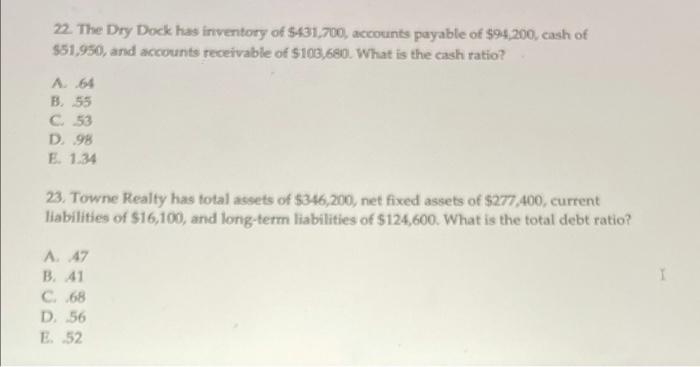# Question need answrrs for both 22. The Dry Dock has inventory of \$431,700, accounts payable of \$94,200, cash of \$51,950, and accounts receivable of \$103,680. What is the cash ratio? A 64 B55 C53 D. 98 E 1.34 23. Towne Realty has total assets of \$346,200, net fixed assets of \$277,400, current liabilities of \$16,100, and long-term liabilities of \$124,600. What is the total debt ratio? A 47 B 41 C 68 D. 56 E 52